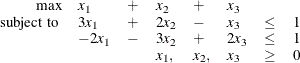## Getting Started: LP Solver

The following example illustrates how you can use the OPTMODEL procedure to solve linear programs. Suppose you want to solve the following problem:You can use the following statements to call the OPTMODEL procedure for solving linear programs:

proc optmodel;
var x{i in 1..3} >= 0;
max f =    x +   x +   x;
con c1:  3*x + 2*x -   x <= 1;
con c2: -2*x - 3*x + 2*x <= 1;
solve with lp / algorithm = ps presolver = none logfreq = 1;
print x;
quit;



The optimal solution and the optimal objective value are displayed in Figure 6.1.

Figure 6.1: Solution Summary

The OPTMODEL Procedure

Problem Summary
Objective Sense Maximization
Objective Function f
Objective Type Linear

Number of Variables 3
Bounded Above 0
Bounded Below 3
Bounded Below and Above 0
Free 0
Fixed 0

Number of Constraints 2
Linear LE (<=) 2
Linear EQ (=) 0
Linear GE (>=) 0
Linear Range 0

Constraint Coefficients 6

Performance Information
Execution Mode Single-Machine
Number of Threads 1

Solution Summary
Solver LP
Algorithm Primal Simplex
Objective Function f
Solution Status Optimal
Objective Value 8

Primal Infeasibility 0
Dual Infeasibility 0
Bound Infeasibility 0

Iterations 5
Presolve Time 0.00
Solution Time 0.05

 x
1 0
2 3
3 5

The iteration log displaying problem statistics, progress of the solution, and the optimal objective value is shown in Figure 6.2.

Figure 6.2: Log

 NOTE: Problem generation will use 4 threads. NOTE: The problem has 3 variables (0 free, 0 fixed). NOTE: The problem has 2 linear constraints (2 LE, 0 EQ, 0 GE, 0 range). NOTE: The problem has 6 linear constraint coefficients. NOTE: The problem has 0 nonlinear constraints (0 LE, 0 EQ, 0 GE, 0 range). NOTE: The LP presolver value NONE is applied. NOTE: The LP solver is called. NOTE: The Primal Simplex algorithm is used. Objective                Entering      Leaving Phase Iteration        Value         Time     Variable      Variable P 1          1    0.000000E+00         0 P 2          2    0.000000E+00         0       x             c2 (S) P 2          3    5.000000E-01         0       x             c1 (S) P 2          4    8.000000E+00         0 P 2          5    8.000000E+00         0 NOTE: Optimal. NOTE: Objective = 8. NOTE: The Primal Simplex solve time is 0.05 seconds.